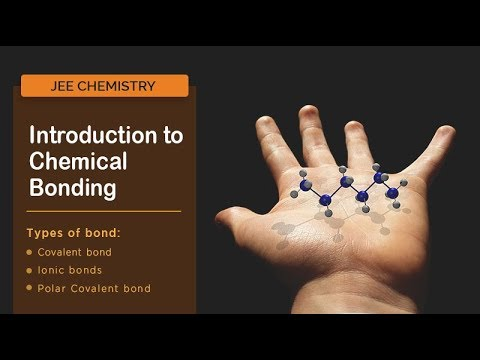Checkout JEE MAINS 2022 Question Paper Analysis : Checkout JEE MAINS 2022 Question Paper Analysis :

# Polar Covalent Bond

In general, a polar bond is a certain class of a covalent bond. We can also say that it is the dividing line between the formation of a pure covalent bond and an ionic bond. However, if we want to define it more accurately, a polar covalent bond is a bond that exists between two atoms consisting of electrons that are unevenly distributed. Due to this state, the molecules tend to have some electrical dipole moment wherein the two ends are either slightly positive or negative.

Electronegativity plays a major role in determining the different types of covalent bonding. Electronegativity is the tendency of an atom to attract a shared pair of an electron towards itself. It has no units simple it is a tendency. The covalent bond formed between two atoms in molecules whose electronegative difference exists is known as a polar covalent bond.

## Explanation of Polar Covalent Bond

Polar covalent bonds are usually formed between two nonmetal atoms having different electronegativities.

Let us consider A and B in which them is electronegativity difference is not equal to zero contains a covalent bond between them. The shared pair of electrons forming a bond between A and B move towards move electronegative B.

Then B gets partial negative charge and attains ‘A’ gets partial positive charge them, with two charges (Poles are formed and it is known as Dipolar molecular or dipole or polar covalent module) as in H – Cl. In this molecule the shared pair of electron moves towards high electronegative chlorine atom. Then H atom gets partial positive charge and Cl atom gets a partial negative charge, hence a dipole is formed.

$$\begin{array}{l}H – Cl \rightarrow H\rightarrow Cl\rightarrow H ^{\delta +} – Cl ^{\delta +}\end{array}$$

## Properties of Polar Covalent Compounds

• Physical state: These compounds can exist as solids due to greater force of interactions.
• Melting and boiling points: These have greater melting and boiling point than non-polar compounds.
• Conductivity: They conduct electricity in the solution state due to the mobility of ions.
• Solubility: These are highly soluble in polar solvents like water.

## Dipole Moment

The polarity of a covalent bond can be explained by a physical quantity called Dipole moment (μ). The dipole moment is defined as the product of charge and distance of separation of charge. The dipole moment is denoted by ‘μ’ and its unit are Debye (or) esu cm.

μ = e × d esu cm

d 🡪 distance between charge or bond length

e 🡪 electronic charge

The net bond dipole moment is

$$\begin{array}{l}\mu =\sqrt{\mu _{1}^{2}+\mu _{2}^{2}+2{{\mu }_{1}}{{\mu }_{2}}\cos \theta }\end{array}$$

Here Ɵ = bond angle.

### Characteristics of Dipole Moment

1. Dipole moment is a vector quantity.

2. Dipole moment is zero for non-polar molecules.

3. For symmetrically applicable molecular dipole moment is = 0.

E.g. 1: Carbon dioxide being linear the net bond moment is equal to zero since the individual bond moment cancel with each other.

E.g. 2: Carbon tetrachloride has zero dipole moment since the molecular is highly symmetrical with tetrahydro structure.

4. Dipole moment is used to calculate the percentage ionic character of a covalent bond.

## Important Questions

1. Define Dipole moment.

Answer: It is the product of charge and distance between the charges.

2. Why polar covalent solids are soluble in water?

Answer: Water has the capacity to break the detractions between the atoms in the molecule hence polar solids are soluble water.

3. What is the main application of Dipole moment?

Answer: Dipole moment represents the bond moment, it helps to calculate percentage ionic character of a covalent bond.

4. Why does co2 have zero dipole moment?

Answer: Co2 ha linear geometry such that bond moments of individual bonds called each other net dipole moment is zero

$$\begin{array}{l}\overset{-m\leftarrow }{\mathop{O}}\,=C\overset{\overset{m}{\mathop{\leftarrow }}\,}{\mathop{=}}\,O\end{array}$$
.

5. The bond length of HCl is 1.27A. Calculate its Dipole moment.

D = 1.27A° = 1.27×10–8 cm

e = 4.8 × 10 –10 esu

μ = e × d

μ = 4.8 ×10–10 × 1.27 × 10 –8

μ = 6.096 × 10–18 esu cm

1 Debye = 10 –18 esu cm

= 6.096 Debye

## Introduction to Chemical Bonding## Chemical Bonding## Frequently Asked Questions – FAQs

### What is a polar covalent bond?

A bond formed between two atoms with a difference in electronegativities by sharing electrons is called a polar covalent bond.

### Give the example of a molecule with a polar covalent bond.

Water (H2O) is a molecule with a polar covalent bond.

### Comment on the melting and boiling points of molecules with polar covalent bonds.

The melting and boiling point of compounds with polar covalent bonds is higher than those of non-polar compounds.

### Do molecules with polar covalent bonds conduct electricity?

Yes, molecules with polar covalent bonds conduct electricity due to the mobility of the ions.

### What is the value of the dipole moment for non-polar molecules?

The dipole moment for non-polar molecules is zero.
Test Your Knowledge on Polar Covalent Bond